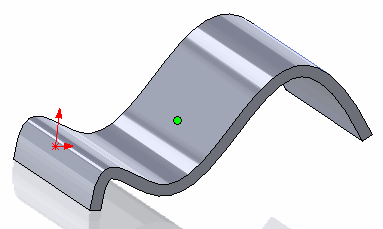Point created at the center of the face

This example demonstrate how to find the parameters (point coordinate and normal) at the center of the face using SOLIDWORKS API. This macro will work with any type of face (planar, cylindrical, toroidal, b-surface etc.)

Center is found as the average of minimum and maximum values of U and V parameters using the ISurface::Evaluate SOLIDWORKS API method.

```Dim swApp As SldWorks.SldWorks
Dim swModel As SldWorks.ModelDoc2

Sub main()

Set swApp = Application.SldWorks

Set swModel = swApp.ActiveDoc

If Not swModel Is Nothing Then

Dim swFace As SldWorks.Face2
Set swFace = swModel.SelectionManager.GetSelectedObject6(1, -1)

If Not swFace Is Nothing Then

Dim vPt As Variant
Dim vNorm As Variant

GetFaceCenterParameters swFace, vPt, vNorm

Debug.Print "Coordinate at face center is: " & vPt(0) * 1000 & ", " & vPt(1) * 1000 & ", " & vPt(2) * 1000
Debug.Print "Normal at face center is: " & vNorm(0) & ", " & vNorm(1) & ", " & vNorm(2)

Else
MsgBox "Please select face"
End If

Else
MsgBox "Please open the model"
End If

End Sub

Sub GetFaceCenterParameters(face As SldWorks.Face2, ByRef point As Variant, ByRef normal As Variant)

Dim vUvBounds As Variant
vUvBounds = face.GetUVBounds

Dim centerU As Double
Dim centerV As Double

centerU = (vUvBounds(0) + vUvBounds(1)) / 2
centerV = (vUvBounds(2) + vUvBounds(3)) / 2

Dim swSurf As SldWorks.Surface
Set swSurf = face.GetSurface

Dim vEvalRes As Variant
vEvalRes = swSurf.Evaluate(centerU, centerV, 0, 0)

Dim dPoint(2) As Double
Dim dNormal(2) As Double

dPoint(0) = vEvalRes(0)
dPoint(1) = vEvalRes(1)
dPoint(2) = vEvalRes(2)

dNormal(0) = vEvalRes(3)
dNormal(1) = vEvalRes(4)
dNormal(2) = vEvalRes(5)

point = dPoint
normal = dNormal

End Sub```